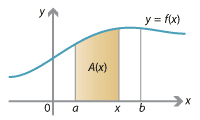## Content

### Calculating areas with antiderivatives

A general method to find the area under a graph $$y=f(x)$$ between $$x=a$$ and $$x=b$$ is given by the following important theorem.

###### Theorem (Fundamental theorem of calculus)

Let $$f(x)$$ be a continuous real-valued function on the interval $$[a,b]$$. Then

$\int_a^b f(x) \; dx = \Big[ F(x) \Big]_a^b = F(b) - F(a),$

where $$F(x)$$ is any antiderivative of $$f(x)$$.

The notation $$\big[ F(x) \big]_a^b$$ is just a shorthand to substitute $$x=a$$ and $$x=b$$ into $$F(x)$$ and subtract; it is synonymous with $$F(b) - F(a)$$.

Note that any antiderivative of $$f(x)$$ will work in the above theorem. Indeed, if we have two different antiderivatives $$F(x)$$ and $$G(x)$$ of $$f(x)$$, then they must differ by a constant, so $$G(x) = F(x) + c$$ for some constant $$c$$. Then we have to get the same answer whether we use $$F$$ or $$G$$, since

\begin{align*} G(b) - G(a) &= \big( F(b) + c \big) - \big( F(a) + c \big)\\ &= F(b) - F(a). \end{align*}

We give a proof of the theorem, assuming our previous statements:

• the derivative of the area function is $$f(x)$$
• any two antiderivatives of $$f(x)$$ differ by a constant.
###### ProofDetailed description of diagram

• As before, let $$A(c)$$ be the area under the graph $$y=f(x)$$ between $$x=a$$ and $$x=c$$. So we have an area function $$A(x)$$ which measures the area between $$a$$ and $$x$$. We must find $$A(b)$$, the area from $$a$$ to $$b$$. The derivative of the area function $$A(x)$$ is $$f(x)$$, so $$A(x)$$ is an antiderivative of $$f(x)$$. As both $$A(x)$$ and $$F(x)$$ are antiderivatives of $$f(x)$$, they must differ by a constant, i.e., $$A(x) = F(x) + K$$ for some constant $$K$$. Since $$A(a) = 0$$, this implies $$F(a) + K = 0$$. Hence $$K = - F(a)$$, and it follows that $$A(x) = F(x) - F(a)$$. Therefore the desired integral is equal to $$A(b) = F(b) - F(a)$$.

$$\Box$$

We can return to our original problem and solve it using the fundamental theorem.

#### Example

Find

$\int_0^5 (x^2 + 1) \; dx.$

#### Solution

An antiderivative of $$x^2 + 1$$ is $$\frac{1}{3} x^3 + x$$, so we have

\begin{align*} \int_0^5 (x^2 + 1) \; dx &= \Bigg[ \frac{1}{3} x^3 + x \Bigg]_0^5\\ &= \Big( \frac{1}{3} \cdot 5^3 + 5 \Big) - \Big( \frac{1}{3} \cdot 0^3 + 0 \Big)\\ &= \frac{125}{3} + 5 = \frac{140}{3} = 46 \frac{2}{3}. \end{align*}
##### Exercise 10

Find

$\int_0^8 (3x^2 + \sqrt{x}) \; dx.$

Next page - Content - Velocity–time graphs